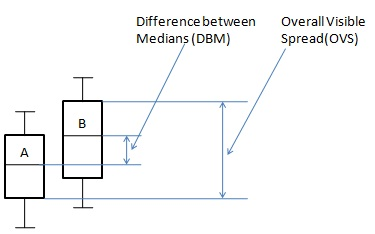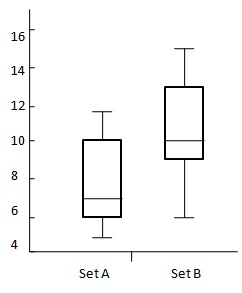# Statistics - Comparing Plots

Groups of population can be compared using box and whisker plots. Overall visible spread and difference between median is used to draw conclusion that there tends to be a difference between two groups or not.## Formula

${P = \frac{DBM}{OVS} \times 100 }$

Where −

• ${P}$ = percentage difference

• ${DBM}$ = Difference Between Medians.

• ${OVS}$ = Overall Visible Spread.

## Rules

• For a sample size of 30 if this percentage is greater than 33% there tends to be a difference between two groups.

• For a sample size of 100 if this percentage is greater than 20% there tends to be a difference between two groups.

• For a sample size of 1000 if this percentage is greater than 10% there tends to be a difference between two groups.

## Example

### Problem Statement

Describe the difference between following sets of data.

Sr.No. Name Set A Set B
1 Max 12 15
2 UQ 10 13
3 Median 7 10
4 LQ 6 9
5 Min 5 6

## Solution

Consider the following diagram −${OVS = 13 - 6 \\[7pt] \ = 7 \\[7pt] \ DBM = 10 -3 \\[7pt] \ = 4 }$

Apply the formula

${P = \frac{DBM}{OVS} \times 100 \\[7pt] \ = \frac{4}{7} \times 100 \\[7pt] \ = 57.14 }$

As percentage is over 33% thus there is difference between Set A and Set B. It is likely that Set B is greater than Set A.

## Useful Video Courses

Video

#### Class 11th Statistics for Economics

40 Lectures 3.5 hours

Video

#### Statistics

40 Lectures 2 hours

Video

#### Applied Statistics in Python for Machine Learning Engineers

66 Lectures 1.5 hours

Video

#### An Introduction to Wait Statistics in SQL Server

22 Lectures 1 hours

Video

#### Geospatial Data Science: Statistics and Machine Learning

60 Lectures 12 hours

Video

#### Basic Statistics & Regression for Machine Learning in Python

65 Lectures 5 hours